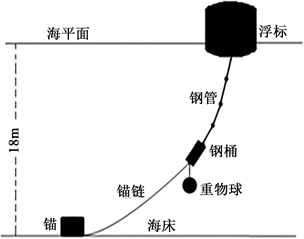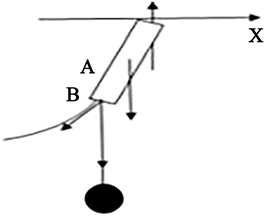# 一类系泊系统的设计问题Design of a Class of Mooring Systems

DOI: 10.12677/APP.2021.111002, PDF, HTML, XML, 下载: 298  浏览: 355

Abstract: The design of mooring system mainly determines the parameter problem of each component of mooring system. In this paper, we take these physical components as a point for force analysis, analyze the relationship between these components, make the whole mooring system in the optimal state under which state each component is in, and list the equilibrium equation according to the static equilibrium condition.

1. 引言Figure 1. Transmission node diagram (structure module diagram only, size ratio not considered)

2. 预备知识

1) 假设钢管之间用铰链链接可自由转动。

2) 假设风是沿平行海平面的方向吹来。

3) 假设锚链和重物球的浮力影响可以忽略。

4) 假设忽略其它原因(如温度，湿度等)对系统影响。

5) 假设浮标不会出现因为风力等因素而被吹翻的情况。

3. 受力分析

${f}_{1x}={f}_{海}$ (3.1)

${f}_{1y}={f}_{浮}-{m}_{1}g$ (3.2)

3.1. 对钢桶受力情况进行分析

$\left({G}_{桶}+{f}_{桶}\right)\cdot {\delta }_{A}+\left({G}_{球}+{f}_{猫}\right)\cdot {\delta }_{B}=0$ (3.3)Figure 2. Steel barrel stress

${\delta }_{A}=\left(-\frac{l}{2}\mathrm{cos}\phi \delta \phi ,\frac{l}{2}\mathrm{sin}\phi \delta \phi \right)$${\delta }_{B}=\left(-l\mathrm{cos}\phi \delta \phi ,l\mathrm{sin}\phi \delta \phi \right)$ 代入上方程(2.3)化简得：

$-\frac{l}{2}{m}_{3}g\mathrm{sin}\phi +\frac{l}{2}{\rho }_{水}{V}_{桶}g\mathrm{sin}\phi +l{f}_{x}\mathrm{cos}\phi -l{m}_{4}g\mathrm{sin}\phi -l{f}_{y}\mathrm{sin}\phi =0$ (3.4)

3.2. 对钢管进行受力分析

$\left(0,-{m}_{2}g+{\rho }_{水}{V}_{管}g\right)\cdot {\delta }_{A}+\left({f}_{21x},{f}_{21y}\right)\cdot {\delta }_{B}=0$ (3.5)

${\delta }_{A}=\left(-\frac{k}{2}\mathrm{cos}{\theta }_{1}\delta {\theta }_{1},\frac{k}{2}\mathrm{sin}{\theta }_{1}\delta {\theta }_{1}\right)$${\delta }_{B}=\left(-k\mathrm{cos}{\theta }_{1}\delta {\theta }_{1},k\mathrm{sin}{\theta }_{1}\delta {\theta }_{1}\right)$ 代入上方程化简得：

$-\frac{k}{2}{m}_{2}g\mathrm{sin}{\theta }_{1}\delta {\theta }_{1}+\frac{k}{2}{\rho }_{水}{V}_{管}g\mathrm{sin}{\theta }_{1}\delta {\theta }_{1}-k{f}_{21x}\mathrm{cos}{\theta }_{1}\delta {\theta }_{1}+k{f}_{21y}\mathrm{sin}{\theta }_{1}\delta {\theta }_{1}=0$ (3.6)

$\left(0,-{m}_{2}g+{\rho }_{水}{V}_{管}g\right)\cdot {\delta }_{A}+\left({f}_{32x},{f}_{32y}\right)\cdot {\delta }_{B}=0$ (3.7)

${\delta }_{A}=\left(-\frac{k}{2}\mathrm{cos}{\theta }_{2}\delta {\theta }_{2},\frac{k}{2}\mathrm{sin}{\theta }_{2}\delta {\theta }_{2}\right)$${\delta }_{B}=\left(-k\mathrm{cos}{\theta }_{2}\delta {\theta }_{2},k\mathrm{sin}{\theta }_{2}\delta {\theta }_{2}\right)$ 代入上方程化简得：

$-\frac{k}{2}{m}_{2}g\mathrm{sin}{\theta }_{2}\delta {\theta }_{2}+\frac{k}{2}{\rho }_{水}{V}_{管}g\mathrm{sin}{\theta }_{2}\delta {\theta }_{2}-k{f}_{32x}\mathrm{cos}{\theta }_{2}\delta {\theta }_{2}+k{f}_{32y}\mathrm{sin}{\theta }_{2}\delta {\theta }_{2}=0$ (3.8)

$\left(0,-{m}_{2}g+{\rho }_{水}{V}_{管}g\right)\cdot {\delta }_{A}+\left({f}_{43x},{f}_{43y}\right)\cdot {\delta }_{B}=0$ (3.9)

${\delta }_{A}=\left(-\frac{k}{2}\mathrm{cos}{\theta }_{3}\delta {\theta }_{3},\frac{k}{2}\mathrm{sin}{\theta }_{3}\delta {\theta }_{3}\right)$${\delta }_{B}=\left(-k\mathrm{cos}{\theta }_{3}\delta {\theta }_{3},k\mathrm{sin}{\theta }_{3}\delta {\theta }_{3}\right)$ 代入上方程化简得：

$-\frac{k}{2}{m}_{2}g\mathrm{sin}{\theta }_{3}\delta {\theta }_{3}+\frac{k}{2}{\rho }_{水}{V}_{管}g\mathrm{sin}{\theta }_{3}\delta {\theta }_{3}-k{f}_{43x}\mathrm{cos}{\theta }_{3}\delta {\theta }_{3}+k{f}_{43y}\mathrm{sin}{\theta }_{3}\delta {\theta }_{3}=0$ (3.10)

$\left(0,-{m}_{2}g+{\rho }_{水}{V}_{管}g\right)\cdot {\delta }_{A}+\left({f}_{4x},{f}_{4y}\right)\cdot {\delta }_{B}=0$ (3.11)

${\delta }_{A}=\left(-\frac{k}{2}\mathrm{cos}{\theta }_{4}\delta {\theta }_{4},\frac{k}{2}\mathrm{sin}{\theta }_{4}\delta {\theta }_{4}\right)$${\delta }_{B}=\left(-k\mathrm{cos}{\theta }_{4}\delta {\theta }_{4},k\mathrm{sin}{\theta }_{4}\delta {\theta }_{4}\right)$ 代入上方程化简得：

$-\frac{k}{2}{m}_{2}g\mathrm{sin}{\theta }_{4}\delta {\theta }_{4}+\frac{k}{2}{\rho }_{水}{V}_{管}g\mathrm{sin}{\theta }_{4}\delta {\theta }_{4}-k{f}_{4x}\mathrm{cos}{\theta }_{4}\delta {\theta }_{4}+k{f}_{4y}\mathrm{sin}{\theta }_{4}\delta {\theta }_{4}=0$ (3.12)

3.3. 对锚链进行受力分析

$\text{d}\left(\mathrm{tan}\theta \right)=\frac{{\rho }_{l}}{H}\text{d}\left({l}_{s}\right)=\frac{{\rho }_{l}}{H}\sqrt{{\left(\text{d}x\right)}^{2}+{\left(\text{d}y\right)}^{2}}=\frac{{\rho }_{l}}{H}\sqrt{1+{\left(\mathrm{tan}\theta \right)}^{2}}\text{d}x$ (3.13)

$\int \frac{d\left(\mathrm{tan}\theta \right)}{\sqrt{1+{\left(tan\theta \right)}^{2}}}=\int \frac{{\rho }_{l}}{H}\text{d}x$ (3.14)

$y=\frac{{\rho }_{l}}{H}ch\left(\frac{{\rho }_{l}}{H}x+{c}_{1}\right)+{c}_{2}$ (3.15)

$y=\frac{{\rho }_{l}}{H}ch\left(\frac{{\rho }_{l}}{H}x+1\right)$ (3.16)

1) 浮标受力情况

2) 钢管受力情况

$\left\{\begin{array}{l}-{f}_{10x}-{f}_{21x}=0\\ -{f}_{10y}-{f}_{21y}-{m}_{2}g+{\rho }_{水}{V}_{管}g=0\end{array}$ ${f}_{21}\mathrm{sin}\left({\theta }_{2}-{\theta }_{1}\right)+\left({\rho }_{水}{V}_{管}g-{m}_{2}g\right)\mathrm{sin}{\theta }_{1}=0$

$\left\{\begin{array}{l}-{f}_{21x}-{f}_{32x}=0\\ -{f}_{21y}-{f}_{32y}-{m}_{2}g+{\rho }_{水}{V}_{管}g=0\end{array}$ ${f}_{32}\mathrm{sin}\left({\theta }_{3}-{\theta }_{2}\right)+\left({\rho }_{水}{V}_{管}g-{m}_{2}g\right)\mathrm{sin}{\theta }_{2}=0$

$\left\{\begin{array}{l}-{f}_{32x}-{f}_{43x}=0\\ -{f}_{32y}-{f}_{43y}-{m}_{2}g+{\rho }_{水}{V}_{管}g=0\end{array}$ ${f}_{43}\mathrm{sin}\left({\theta }_{4}-{\theta }_{3}\right)+\left({\rho }_{水}{V}_{管}g-{m}_{2}g\right)\mathrm{sin}{\theta }_{3}=0$

$\left\{\begin{array}{l}-{f}_{43x}-{f}_{54x}=0\\ -{f}_{43y}-{f}_{54y}-{m}_{2}g+{\rho }_{水}{V}_{管}g=0\end{array}$ ${f}_{54}\mathrm{sin}\left({\theta }_{5}-{\theta }_{4}\right)+\left({\rho }_{水}{V}_{管}g-{m}_{2}g\right)\mathrm{sin}{\theta }_{4}=0$

$\left\{\begin{array}{l}-{f}_{54x}-{f}_{65x}=0\\ -{f}_{54y}-{f}_{65y}-{m}_{3}g-{m}_{4}g+{\rho }_{水}{V}_{桶}g=0\end{array}$

$L=h+l\left(\mathrm{cos}{\theta }_{1}+\mathrm{cos}{\theta }_{2}+\mathrm{cos}{\theta }_{3}+\mathrm{cos}{\theta }_{4}\right)+{l}_{t}\mathrm{cos}{\theta }_{5}+{s}_{y}$ (3.17)

4. 总结与展望

  王丹, 刘家新. 一般状态下悬链线方程的应用[J]. 船海工程, 2007, 36(3): 26-28.  袁梦, 范菊, 朱仁传, 缪国平. 基于悬链线理论的系泊系统势能[J]. 上海交通大学学报, 2011, 45(4): 597-603.  康健, 陈国明, 朱本瑞. 四锚链工程船舶锚泊优化分析[J]. 石油矿场机械, 2013, 42(2): 63-66.  范会渠. 弹性悬链线方程参数变换法及其工程应用[J]. 力学与实践, 2010, 32(2): 32-35.  郭迎雪, 姚晴. 基于悬链线方程的系泊系统状态分析[J]. 应用数学进展, 2017, 6(3): 296-307.  刘涌国. 带悬锤的悬链线方程求解及其简化方法[J]. 水运工程, 2014(3): 103-106.  刘阳, 李杨, 闫琛. 悬链锚桩系泊系统锚桩结构设计分析[J]. 海洋工程装备与技术, 2019, 6(1): 199.  何英豪, 朱雨薇, 韩冰凌. 基于悬链线方程的系泊系统分析[J]. 工业设计, 2016(11): 136-137, 139.  王希春, 王静. 利用自悬链模型研究系泊系统的设计问题[J]. 数学学习与研究, 2018(13): 139.  孙宁松. 海上移动式平台锚泊定位系统锚索链受力分析[J]. 中国海洋平台, 1997, 23(2): 41-44.  张继明, 范秀涛. 海洋资料浮标锚泊系统的系泊力计算[J]. 山东科学, 2014, 27(2): 19-24.  王领, 刘生法. 深水大型浮台系泊系统水动力计算分析[J]. 广东造船, 2018(5): 16-18.  洪文渊. 绷紧式深海系泊缆与上部浮体的耦合动力分析[D]: [硕士学位论文]. 杭州: 中国计量学院, 2015.  潘斌, 高婕. 浮标系泊系统静力计算[J]. 重庆交通学院学报, 1997, 16(1): 68-73.  谭兵, 伍悦. 基于迭代搜索算法的系泊系统的优化设计[J]. 兰州文理学院学报, 2017, 31(5): 31-35, 45.  刘昌欣, 范兴奎. 基于静力学分析的系泊系统状态浅析[J]. 应用数学进展, 2017, 6(3): 357-366.  王宏伟. 深海系泊系统模型截断技术研究[D]: [博士学位论文]. 哈尔滨: 哈尔滨工程大学, 2011.  郑中强. 海洋系泊系统的非线性动力学研究[D]: [博士学位论文]. 青岛: 中国海洋大学, 2015.  张欣欣, 郭小天, 张亮. 非线弹性索对系泊系统性能的影响[J]. 应用科技, 2014(1): 84-88.  张隆辉. 系泊系统主动式截断混合模型试验技术仿真研究[D]: [硕士学位论文]. 北京: 中国舰船研究院, 2016.  王贝贝, 于蓉, 马灵灵, 周克元. 系泊系统的最优设计[J]. 保山学院学报, 2017(5): 41-43.  李斐, 吕文, 李琴. 水流影响下系泊系统设计的数学模型[J]. 烟台大学学报, 2017, 30(3): 173-179.  成海亮. 船舶T型系泊系统安全性研究[J]. 中国水运(下半月), 2017, 17(6): 22-23.  张路莹, 刘绍庆. 基于多目标规划的系泊系统的设计[J]. 曲阜师范大学学报, 2017, 43(3): 49-53.  吴婷婷. 开敞式码头系泊系统状态预测方法研究[D]: [硕士学位论文]. 大连: 大连交通大学, 2016.  徐剑峰. 浅水斜底地形下超大型浮体单模块系泊系统研究[D]: [硕士学位论文]. 上海: 上海交通大学, 2018.  尘颖, 许佰雁. 基于迭代法的系泊系统问题模型分析[J]. 洛阳师范学院学报, 2017, 36(2): 19-22.  袁培银, 赵宇. 多浮体式系泊系统设计及水动力性能分析[J]. 舰船科学技术, 2016, 38(2): 80-84.